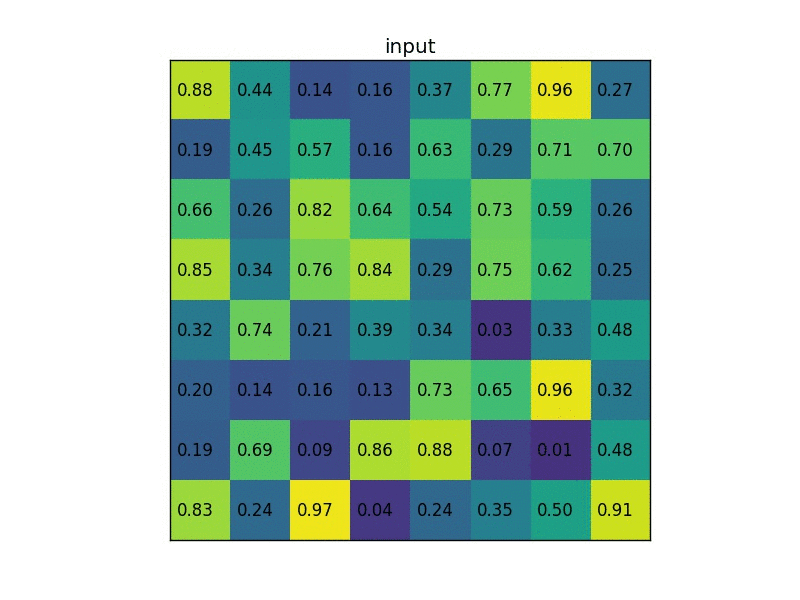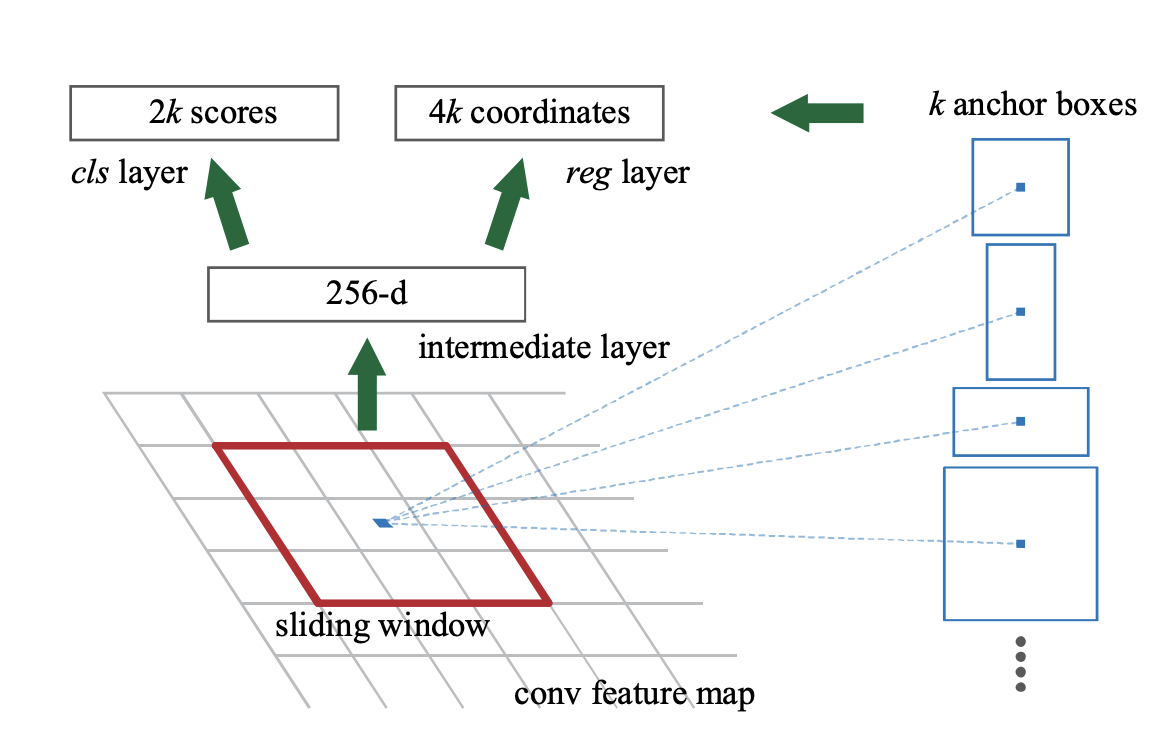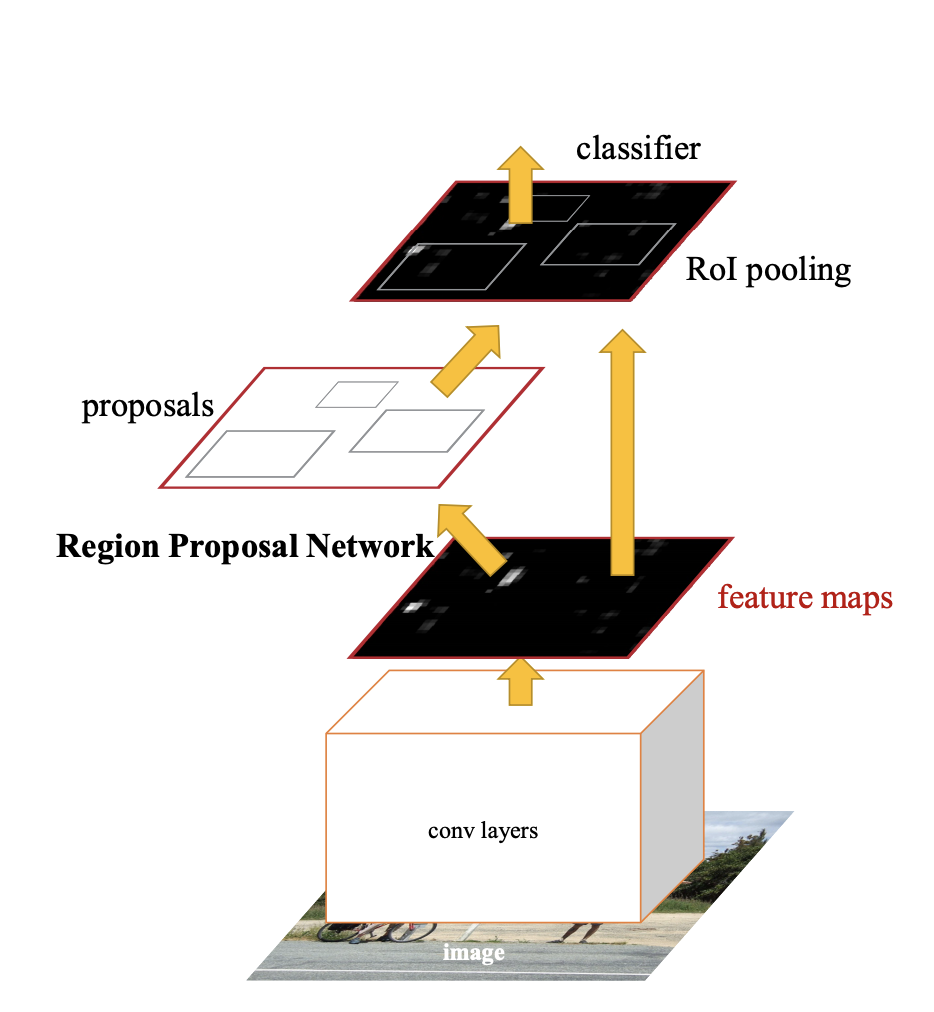# “Faster RCNN --- Towards Real-Time Object Detection with Region Proposal Networks”

## ROI pooling and ROI alignment:

1. an RoI is a rectangular window into a conv feature map. Each RoI is defined by a four-tuple (r, c, h, w) that specifies its top-left corner (r, c) and its height and width (h, w).
2. It is a type of pooling layer which performs max pooling on inputs (here, convnet feature maps) of non-uniform sizes and produces a small feature map of fixed size (say 7x7). The choice of this fixed size is a network hyper-parameter and is predefined
3. The purpose for this specific design is that it will computation much faster.## Region Proposal Networks :

1. A Region Proposal Network (RPN) takes an image (of any size) as input and outputs a set of rectangular object proposals, each with an objectness score
2. goal is to share computation with a Fast R-CNN object detection network
3. To generate region proposals, slide a small network over the convolutional feature map output by the last shared convolutional layer## Anchors:

1. At each sliding-window location, we simultaneously predict multiple region proposals, where the number of maximum possible proposals for each location is denoted as k. So the reg layer has 4k outputs encoding the coordinates of k boxes, and the cls layer outputs 2k scores that estimate probability of object or not object for each proposal
2. The k proposals are parameterized relative to k reference boxes–anchors
3. An anchor is centered at the sliding window in question, and is associated with a scale and aspect ratio . By default we use 3 scales and 3 aspect ratios, yielding k = 9 anchors at each sliding position
4. An important property of our approach is that it is translation invariant

## Multi-Scale Anchors as Regression References:

1. there have been two popular ways for multi-scale predictions. The first way is based on image/feature pyramids(useful but time-consuming)
2. The second way is to use sliding windows of multiple scales (and/or aspect ratios) on the feature maps.
3. our anchor-based method is built on a pyramid of anchors, which is more cost-efficient. Our method classifies and regresses bounding boxes with reference to anchor boxes of multiple scales and aspect ratios. It only relies on images and feature maps of a single scale, and uses filters (sliding windows on the feature map) of a single size

## Loss Function

1. We assign a positive label to two kinds of anchors: (i) the anchor/anchors with the highest Intersection-overUnion (IoU) overlap with a ground-truth box, or (ii) an anchor that has an IoU overlap higher than 0.7 with 5 any ground-truth box.
\begin{aligned} L\left(\left\{p_{i}\right\},\left\{t_{i}\right\}\right) &=\frac{1}{N_{c l s}} \sum_{i} L_{c l s}\left(p_{i}, p_{i}^{*}\right) \\+\lambda & \frac{1}{N_{r e g}} \sum_{i} p_{i}^{*} L_{r e g}\left(t_{i}, t_{i}^{*}\right) \end{aligned}

2. $t_{i}$ is a vector representing the 4 parameterized coordinates of the predicted bounding box;The ground-truth label $p^{∗}_{i}$ is 1 if the anchor is positive, and is 0 if the anchor is negative

## Bounding box regression:

1. from above, the parameterization of t can be described as follows: \begin{aligned} t_{\mathrm{x}} &=\left(x-x_{\mathrm{a}}\right) / w_{\mathrm{a}}, \quad t_{\mathrm{y}}=\left(y-y_{\mathrm{a}}\right) / h_{\mathrm{a}} \\ t_{\mathrm{w}} &=\log \left(w / w_{\mathrm{a}}\right), \quad t_{\mathrm{h}}=\log \left(h / h_{\mathrm{a}}\right) \\ t_{\mathrm{x}}^{*} git&=\left(x^{*}-x_{\mathrm{a}}\right) / w_{\mathrm{a}}, \quad t_{\mathrm{y}}^{*}=\left(y^{*}-y_{\mathrm{a}}\right) / h_{\mathrm{a}} \\ t_{\mathrm{w}}^{*} &=\log \left(w^{*} / w_{\mathrm{a}}\right), \quad t_{\mathrm{h}}^{*}=\log \left(h^{*} / h_{\mathrm{a}}\right) \end{aligned}

## Put it together## YONG HUANG

Hi, I'm Yong Huang. I am a Ph.D. student in Computer Science at UC Irvine, I am interested in machine learning for healthcare, before coming to UC Irvine, I did my Master at Cornell Tech and did my undergrad at Tongji University. Thank you for visiting my site. I recently removed several blogs because I found mathjax is broken, sorry about the incovenience.